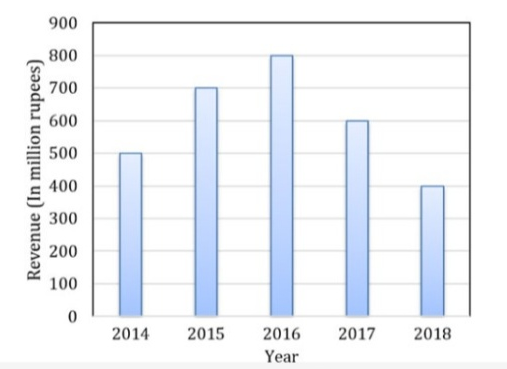# GATE | GATE CS 2020 | Question 10

The total revenue of a company during 2014−2018 is shown in the bar graph. If the total expenditure of the company in each year is 500 million rupees, then the aggregate profit or loss (in percentage) on the total expenditure of the company during 2014−2018 is _________ .(A) 16.67% profit
(B) 16.67% loss
(C) 20% profit
(D) 20% loss

Explanation: Bar-graph: 2014-2018,

Total expenditure,

```= 500 million/year
= 500 × 5
= 2500 million ```

Now, Revenue total (from the graph),

```= 400 + 500 + 600 + 700 + 800
= 3000 million ```

Therefore, Profit,

`= 3000 - 2500 = 500 `

Profit (in percentage),

```= (500/2500)*100 %
= 20 % profit ```

Option (C) is correct.

Quiz of this Question

My Personal Notes arrow_drop_up
Article Tags :

Be the First to upvote.

Please write to us at contribute@geeksforgeeks.org to report any issue with the above content.